NCERT Exemplar Class 6 Science Chapter 12 Electricity and Circuits are part of NCERT Exemplar Class 6 Science. Here we have given NCERT Exemplar Class 6 Science Solutions Chapter 12 Electricity and Circuits.

## NCERT Exemplar Class 6 Science Solutions Chapter 12 Electricity and Circuits

Multiple Choice Questions

Question 1.
Choose from the options a, b, c and d given in the figure which shows the correct direction of current .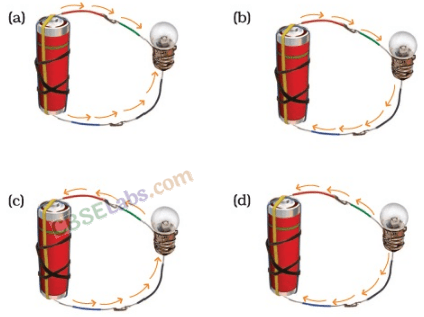Solution:
(b): In an electric circuit, the direction of current is taken to be from the positive terminal to the negative terminal of the electric cell.

Question 2.
Choose the incorrect statement.
(a) A switch is the source of electric current in a circuit.
(b) A switch helps to complete or break the circuit.
(c) A switch helps us to use electricity as per our requirement.
(d) When the switch is open there is an air gap between its terminals.
Solution:
(a) : An electric cell is the source of electric current in a circuit.

Question 3.
In an electric bulb, light is produced due to the glowing of
(a) the glass case of the bulb
(b) the thin filament
(c) the thick wires supporting the filament
(d) gases inside glass case of the bulb.
Solution:
(b)

Question 4.
In the following arrangement shown in figure, the bulb will not glow if the ends A and B are connected with(a) A steel spoon
(b) A metal clip
(c) A plastic clip
(d) A copper wire
Solution:
(c): Plastic clip is an insulator. It does not conduct electricity.

Question 5.
In the circuit shown in figure, when the switch is moved to’ON’ position,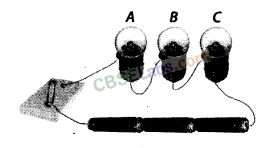(a) the bulb A will glow first
(b) the bulb B will glow first
(c) the bulb C will glow first
(d) all bulbs will glow together.
Solution:
(d): As soon as the circuit gets completed, current is found at every point in the circuit instantly.

Question 6.
Filament of a torch bulb is
(a) a metal case
(b) metal tip at the centre of the base
(c) two thick wires
(d) a thin wire.
Solution:
(d)

Question 7.
Paheli is running short of connecting wires. To complete an electric circuit, she may use a
(a) glass bangle
(c) rubber pipe
(d) steel spoon.
Solution:
(d): Steel spoon is a good conductor of electricity.

Question 8.
In which of the following circuits A, B and C given in figure, the cell will be used up very rapidly?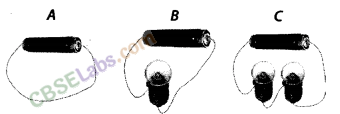Solution:
In circuit A, the cell will be used up very rapidly.

Question 9.
Figure shows a bulb with its different parts marked as 1,2,3,4 and 5. Which of them label the terminals of the bulb?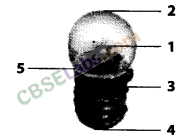Solution:
Labels 3 and 4 are the terminals of the bulb.

Question 10.
You are provided with a bulb, a cell, a switch and some connecting wires. Draw a diagram to show the connections between them to make the bulb glow.
Solution:Question 11.
Will the bulb glow in the circuit shown in figure ? Explain.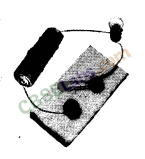Solution:
No, the bulb will not glow in this circuit because the switch is open and the circuit is incomplete. Current flows only in a closed circuit.

Question 12.
An electric bulb is connected to a cell through a switch as shown in figure. When the switch is brought in ‘ON’ position, the bulb does not glow. What could be the possible reason/s for it? Mention any two of them.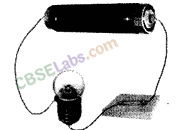Solution:
There could be following reasons for it:
(i) Cell is a used one.
(ii) Bulb is fused.
(iii) Loose connections.
(iv) Break in connecting wires.

Question 13.
A torch requires 3 cells. Show the arrangement of the cells, with a diagram, inside the torch so that the bulb glows.
Solution: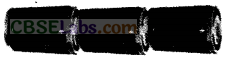Question 14.
When the chemicals in the electric cell are used up, the electric cell stops producing electricity. The electric cell is then replaced with a new one. In case of rechargeable batteries (such – as the type used in mobile phones, camera and inverters), they are used again and again. How?
Solution:
Rechargeable batteries can be recharged • by providing them appropriate current. These are called secondary cells or storage cells.

Question 15.
Paheli connected two bulbs to a cell as shown f in figure,She found that filament of bulb B is broken. Will the bulb A glow in this circuit? Give reason.
Solution:
No, the bulb will not glow in this circuit as the filament of bulb B is broken. So the circuit remains incomplete.

Question 16.
Why do bulbs have two terminals?
Solution:
Bulb consists of a tiny thin wire called filament. Bulb has two terminals to connect the filament within the circuit so that current can pass through it.

Question 17.
Which of the following arrangement A, B, C and D given in figure should not be set up? Explain, why?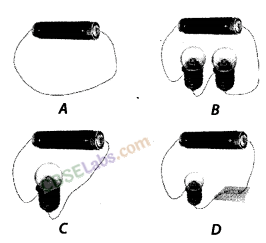Solution:
Arrangement A is not desirable and should not be set up. This will exhaust the cell very quickly as large current will flow through it.

Question 18.
A fused bulb does not glow. Why?
Solution:
In a fused bulb, the filament is broken and the circuit is incomplete.

Question 19.
Paheli wanted to glow a torch bulb using a cell. She could not get connecting wires, instead, she got two strips of aluminium foil. Will she succeed? Explain, how?
Solution:
Yes, she will succeed as aluminium foil strips can act as connecting wires being good conductor of electricity.

Question 20.
Boojho has a cell and a single piece’ of connecting wire. Without cutting the wire in two, will he be able to make the bulb glow? Explain with the help of a circuit diagram.
Solution:
Yes, using the arrangement given in figure, he can succeed in getting the bulb glow.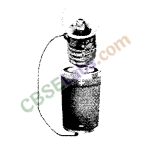He can connect second terminal of the bulb directly to the positive terminal of the cell as given in figure. In this way, circuit will get completed without using another piece of connecting wire.

Question 21.
Figure A and B, show a bulb connected to a cell in two different ways.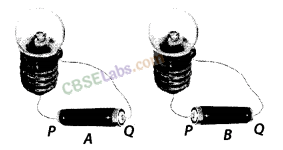(i) What will be the direction of the current through the bulb in both the cases (Q to P orPto Q)?
(ii) Will the bulb glow in both the cases?
(iii) Does the brightness of the glowing bulb depend on the direction of current through it?
Solution:
(i) In case A: Q toP
In case B: P to Q
(ii) Yes, the bulb will glow in both the cases as the circuit is complete in both the cases.
(iii) No, the brightness of the glowing bulb does not depend on the direction of current through it.

Question 22.
Think of six activities which use electric current. Also name the devices used to perform the activity.
Example:

 Activity you perform get light Device Torch

Solution:

 Activity you perform Device (i) Get light Torch (ii) Heat water Geyser/ immersion rod (iii) Make toast Toaster (iv) Cook food Heater/microwave (v) Listen to music CD player/ radio/i-pod (vi) Watch movies TV/Laptop

Question 23.
A torch is not functioning, though contact points in the torch are in working condition. What can be the possible reasons for this? Mention any three.
Solution:
The possible reasons could be
(i) the bulb may be fused.
(ii) the cells may have been used up.
(iii) the cells are not placed in the correct order.
(iv) the switch is faulty.

We hope the NCERT Exemplar Class 6 Science Chapter 12 Electricity and Circuits will help you. If you have any query regarding NCERT Exemplar Class 6 Science Solutions Chapter 12 Electricity and Circuits, drop a comment below and we will get back to you at the earliest.